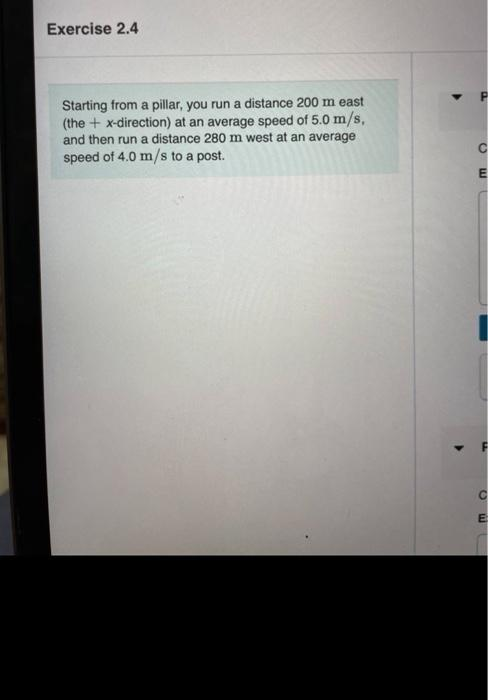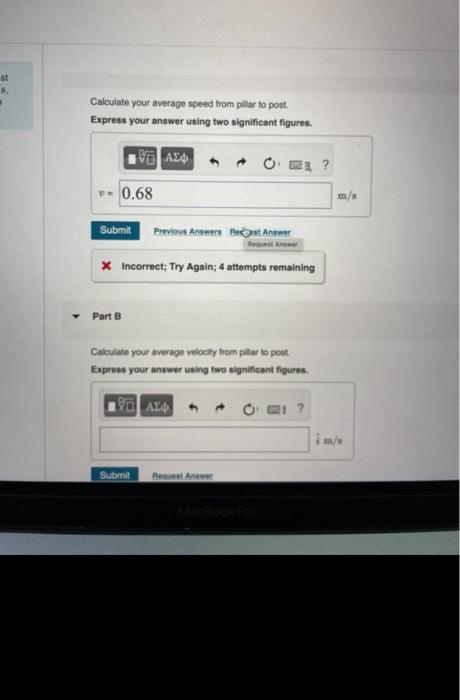# Question Solved1 AnswerExercise 2.4 Starting from a pillar, you run a distance 200 m east (the + x-direction) at an average speed of 5.0 m/s, and then run a distance 280 m west at an average speed of 4.0 m/s to a post. C E F с E st Calculate your average speed from pillar to post. Express your answer using two significant figures. ΑΣΦ - 0.68 Submit Previous Answer Det Answer * Incorrect; Try Again; 4 attempts remaining Part B Calculate your average velocity from pilar to post. Express your answer using two significant figures Η ΑΣΦ ? Submit Request ATranscribed Image Text: Exercise 2.4 Starting from a pillar, you run a distance 200 m east (the + x-direction) at an average speed of 5.0 m/s, and then run a distance 280 m west at an average speed of 4.0 m/s to a post. C E F с E st Calculate your average speed from pillar to post. Express your answer using two significant figures. ΑΣΦ - 0.68 Submit Previous Answer Det Answer * Incorrect; Try Again; 4 attempts remaining Part B Calculate your average velocity from pilar to post. Express your answer using two significant figures Η ΑΣΦ ? Submit Request A
More
Transcribed Image Text: Exercise 2.4 Starting from a pillar, you run a distance 200 m east (the + x-direction) at an average speed of 5.0 m/s, and then run a distance 280 m west at an average speed of 4.0 m/s to a post. C E F с E st Calculate your average speed from pillar to post. Express your answer using two significant figures. ΑΣΦ - 0.68 Submit Previous Answer Det Answer * Incorrect; Try Again; 4 attempts remaining Part B Calculate your average velocity from pilar to post. Express your answer using two significant figures Η ΑΣΦ ? Submit Request A